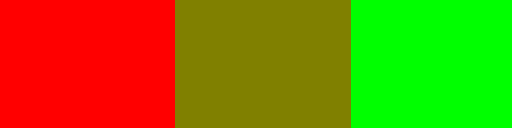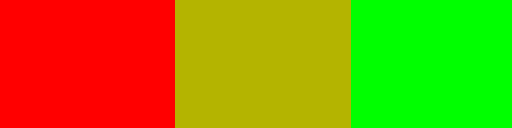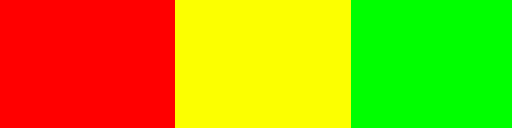# dynamic weather day night cycle

its been a while,

I working on an simulation for weather (rainy, sunny, misty) and the different day times

for example: rainy day at 3pm

and now looking for some dynamic ‘color changers’ I try the lerp function:

``````
def smooth_color_change(new_color, old_color, speed)
return old_color.lerp(new_color, speed)

note: not the code I use just for demonstrate

``````

but lerp is way to impacting/slowing down

also trying:

``````
past_color, present_color, day_time = bge.time.dayTimeState()
diff = [n-d for n,d in zip(past_color, present_color)]
start = day_time
stop = day_time

x = ((bge.time.hour+bge.time.minute/60)/24)
y = ((bge.time.hour+bge.time.minute/60)/start)
z = ((bge.time.hour+bge.time.minute/60)/stop)

a = y/z
e = x*a

r = past_color - diff*e
g = past_color - diff*e
b = past_color - diff*e
bgl.glClearColor(r, g, b, 1)
bgl.glClear(bgl.GL_COLOR_BUFFER_BIT)

``````

but that didn’t work!

so I need some ideas how to solve this problem

Zu erst, guten tag :)…tut mir leid mein deutsch ist sehr schlecht.
are you changing the sky color or the color of an object? I find that lerping on my system also seems to be wasteful…it is faster for me to just change my sky domes using replace mesh across multiple meshes, or animating the color with an action actuator…

as for a faster way to accomplish this in code…I would be very interested in seeing if someone has a better method…lerping would seem to be practical…but as we have already discussed it is slow.

Lerping colour is a funny business. If you simply lerp each value you get some funny results. For instance if I lerp [1, 0, 0] with [0, 1, 0] (red with green) with a factor of 0.5 you end up with [0.5, 0.5, 0] which is yellow - but it’s a dark yellow which isn’t visually “halfway between.”Technically it’s halfway between the colours, but it doesn’t look like it visually. As such, we cpuld do a spherical lerp (slerp) which ends up with [0.707, 0.707, 0] as our midpoint. This looks a bit better, but the plain red and plain green still look brighter:Or we can decompose into HSV and lerp the HSV, which gives us the halfway between red and green as … [1, 1, 0]:This preserves luminosity and saturation.

Hopefully this gives you an idea why lerping colours is more processor intensive than you may expect. It has to be converted into HSV, lerped, and then converted back into RGB.

---- edit ----
Actually, I’m wrong. It probably doesn’t do HSV lerp, instead it uses another colour space called HCL, which is even more expensive to convert.

thanks both of you for helping:

method from an older blog but a bit polished by me:

``````
night = [0.007895, 0.009149, 0.015995]
day = [0.407240, 0.496933, 0.658375]

diff = [n-d for n,d in zip(night, day)]

a = 12
b = 0.5
c = math.pi
t = ((bge.time.hour+bge.time.minute/60))*((15*math.pi/180*2))
y = (math.cos(c-t*b)*a)

if y &gt; 1:
y = 1
elif y &lt; 0:
y = 0

r = night - diff*y
g = night - diff*y
b = night - diff*y
bgl.glClearColor(r, g, b, 1)
bgl.glClear(bgl.GL_COLOR_BUFFER_BIT)

``````

colors are just for testings but if I want colors for each weather typ it seams a bit usless
maybe I have to define more than just two points (old color, new color)

If you can lerp twp colours, you can lerp “n” colours. It’s just a case of where the colour-space conversion takes place.

If we have ten different colours, then we need ten values to represent how much of each to use. In order to ensure we don’t end up with “too much” mixing, we need the vector representing how much of each to be normalized. This prevents the case of having two colours “active” at the same time.

With a plain linear lerp this would be easy as you could throw all your colours into a matrix, and do the lerp using linear algebra. However, we can do something similar by converting to the colour space before the lerp. I’m going to approximate with an HSV lerp here.

Example:

``````import mathutils

COLORS = [
[1, 0, 0], # Red
[0, 1, 0], # Green
[0, 0, 1], # Blue
[1, 1, 0], # Yellow
[1, 0, 1], # Pink
]

def to_conv_space(col):
'''Convert into the color space used to lerping. In this case, hsv'''
return mathutils.Vector(mathutils.Color(col).hsv)

def from_conv_space(col):
'''Convert from the color space used for lerping back into rgb'''
out = mathutils.Color()
out.v = col
out.s = col # Always set saturation before hue otherwise hue may be discarded
out.h = col
return out

def normalize(vec):
'''Manhatten normalize so that the vector so that the sum is 1'''
vec_len = sum(v for v in vec)
return [v/vec_len for v in vec]

def matrix_multiply(mat, vec):
'''Multiplies a matrix by a vector. Matrix columns must match vector length'''
individual_values = [c*vec[i] for i, c in enumerate(mat)]
return sum(individual_values, mathutils.Vector())

hsv_colors = [to_conv_space(c) for c in COLORS] # Only needs to run at game start.

# How much of each color we want. This should be normalized on the nth norm.
# clearly this vector isn't because we've specified both red and green
our_choice = [1, 1, 0, 0, 0]

our_choice = normalize(our_choice)
# [0.5, 0.5, 0, 0, 0] - whew, no more "extra" colors.
# We are aiming for half-way between the first two colours, in this case
# halfway between red and green.

# muliply the color matrix, which is effectively our "lerp"
out_color_in_conv_space = matrix_multiply(hsv_colors, our_choice)

# Convert back into RGB
out_color_in_rgb = from_conv_space(out_color_in_conv_space)

print(out_color_in_rgb)
# [1, 1, 0]  which is yellow, which is halfway between red and green in HSV-space

``````

Because we only need to do the inverse colour transform once (assuming the set of colours doesn’t change), this method should be able to be made reasonably fast. It would be even faster if we did our matrix math inside numpy - but I have yet to learn the numpy API.

thanks for the example
what about using not rgb but hsv instead so i didn’t need to convert?
How about individual timing?
example:

sunny day at 12am --to–> rain fall at 1pm
bright day dark day

also not shure about ‘scene.pre_draw.append(myfunction_above)’

Yes, if you worked everything in HSV at the start then you can skip some of the conversion steps. (although you still need to go to RGB at the end to apply it to the objects).

So what I did was to define a way to mix colours. To figure out what mix you need when is a far harder problem (at least for my not-very-artistic mind). I can’t see many easy ways to do this. You could define a bunch of (mathematical) functions to define when each color has to appear. For example if you have colors for day, night, sunset, sunrise, then you need to somehow control all of these (generate the “our_choice” list in my example). The sunny/sunset/sunrise/night can be completely time based as a bunch of (mathematical) triangular functions. You could define each of these four times for each weather condition (eg clear, cloudy, rainy, snowy). Then you can mix between the weather conditions. I envisage this as coming down to a single (python) function that takes the time of day and the weather as an input. The weather would likely be a vector or a list so that you can blend between different weathers.

The scene.pre_draw just runs a function every frame. (in that case, the “myfunction_above” function)